# 罗曼蒂克消亡史_挑子学习笔记：DBSCAN算法的python实现

DBSCAN（Density-Based Spatial Clustering of Applications with Noise）聚类算法，是一种基于高密度连通区域的、基于密度的聚类算法，能够将具有足够高密度的区域划分为簇(Cluster)，并在具有噪声的数据中发现任意形状的簇。DBSCAN算法通过距离定义出一个密度函数，计算出每个样本附近的密度，从而根据每个样本附近的密度值来找出那些样本相对比较集中的区域，这些区域就是我们要找的簇。

# 1. DBSCAN算法的基本原理

DBSCAN算法中有两个重要参数：\$varepsilon\$表示定义密度时的邻域半径，\$M\$ 表示定义心点时的阈值。

1. \$varepsilon\$邻域

egin{equation*}
N_{varepsilon}(x) = { y in X: d(y,x) le varepsilon }
end{equation*}

egin{equation*}
N_{varepsilon}(i) = { j: d(y^{(j)},x^{(i)}) le varepsilon; ; y^{(j)},x^{(i)} in X }
end{equation*}

2. 密度

3. 核心点

4. 边界点

5. 噪声点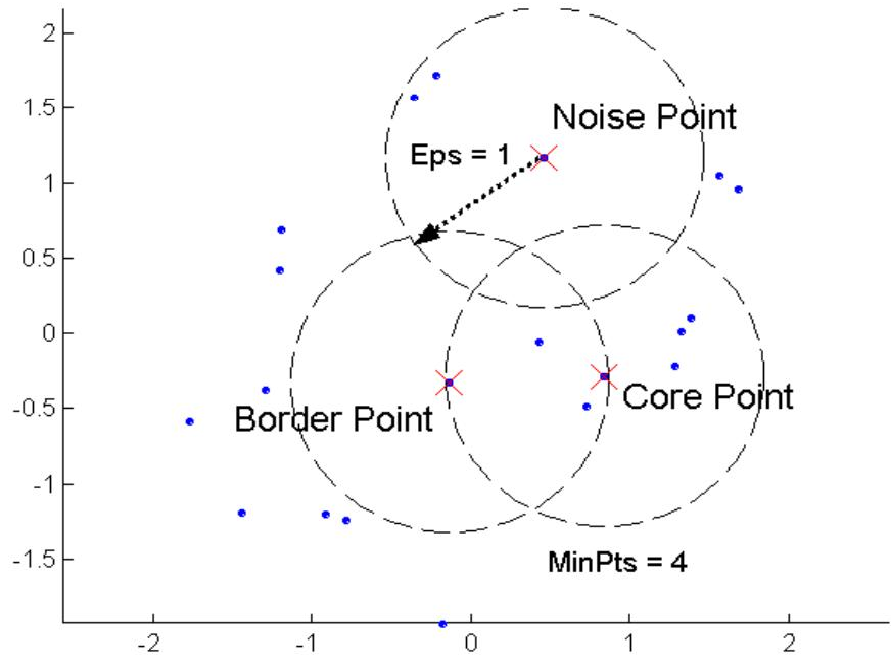6. 直接密度可达

7. 密度可达

7.1. 当\$m = 2\$时，密度可达即为直接密度可达。实际上，密度可达是直接密度可达的传递闭包。

7.2. 密度可达关系不具有对称性。若\$p^{(m)}\$是从\$p^{(1)}\$密度可达的，那么\$p^ {(1)}\$ 不一定是从\$p^{(m)}\$密度可达的。根据上述定义可知，\$p^{(1)}, p^{(2)}, ..., p^{(m-1)}\$必须为核心点，而\$p^{(m)}\$可以是核心点，也可以是边界点。当\$p^ {(m)}\$是边界点时，\$p^{(1)}\$一定不是从\$p^{(m)}\$密度可达的。

8. 密度相连

9. 簇（cluster）

• 若\$x in C\$，且\$y\$是从\$x\$密度可达的，则\$y in C\$，
• 若\$x in C\$，\$y in C\$，则\$x,y\$是密度相连的。

DBSCAN 算法基于以下一个基本事实：对于任一核心点\$x\$，数据集\$X\$中所有从\$x\$ 密度可达的数据点可以构成一个完整的簇\$C\$，且\$x in C\$。其核心思想描述如下:从某个选定的核心点出发，不断向密度可达的区域扩张，从而得到一个包含核心点和边界点的最大化区域，区域中任意两点密度相连。

# 2.DBSCAN算法的实现

《数据挖掘概念与技术》给出的算法伪代码如下: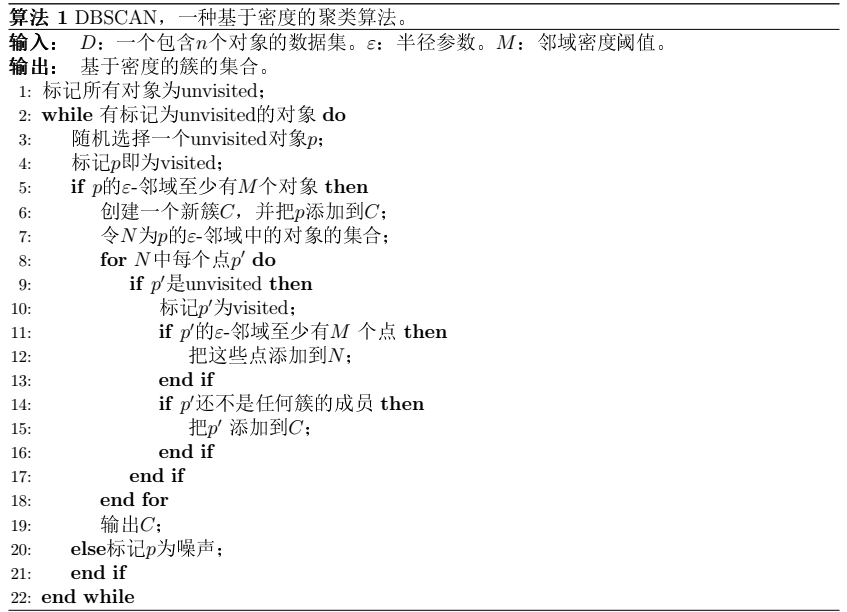egin{equation*}
m_i =
egin{cases}
j, & ext{若}x^{(i)} ext{属于第}j ext{个簇}; \
-1, & ext{若}x^{(i)} ext{为噪声点}
end{cases}
end{equation*}
DBSCAN算法的目标就是生成标记数组\$m_i, ; i=1,...,n\$.

``` 1 # visitlist类用于记录访问列表
2 # unvisitedlist记录未访问过的点
3 # visitedlist记录已访问过的点
4 # unvisitednum记录访问过的点数量
5 class visitlist:
6     def _init_(self, count=0):
7         self.unvisitedlist=[i for i in range(count)]
8         self.visitedlist=list()
9         self.unvisitednum=count
10
11     def visit(self, pointId):
12         self.visitedlist.append(pointId)
13         self.unvisitedlist.remove(pointId)
14         self.unvisitednum -= 1```

DBSCAN算法实现代码如下:

``` 1 import numpy as np
2 import matplotlib.pyplot as plt
3 import math
4 import random
5
6 def  dist(a, b):
7     # 计算a,b两个元组的欧几里得距离
8     return math.sqrt(np.power(a-b, 2).sum())
9
10 def my_dbscanl(dataSet, eps, minPts):
11     # numpy.ndarray的 shape属性表示矩阵的行数与列数
12     nPoints = dataSet.shape
13     # (1)标记所有对象为unvisited
14     # 在这里用一个类vPoints进行买现
15     vPoints = visitlist(count=nPoints)
16     # 初始化簇标记列表C,簇标记为 k
17     k = -1
18     C = [-1 for i in range(nPoints)]
19     while(vPoints.unvisitednum > 0):
20         # (3)随机上选择一个unvisited对象p
21         P = random.choice(vPoints.unvisitedlist)
22         # (4)标记p为visited
23         vPoints.visit(p)
24         # (5)if p的\$varepsilon\$-邻域至少有MinPts个对象
25         # N是p的\$varepsilon\$-邻域点列表
26         N = [i for i in range(nPoints) if dist(dataSet[i], dataSet[p])<= eps]
27         if  len(N) >= minPts:
28             # (6)创建个新簇C，并把p添加到C
29             # 这里的C是一个标记列表，直接对第p个结点进行赋植
30             k += 1
31             C[p]=k
32             # (7)令N为p的-邻域中的对象的集合
33             # N是p的\$varepsilon\$-邻域点集合
34             # (8) for N中的每个点p"
35             for p1 in N:
36                 # (9) if p"是unvisited
37                 if p1 in vPoints.unvisitedlist:
38                     # (10)标记p’为visited
39                     vPoints.visit(p1)
40                     # (11) if p"的\$varepsilon\$-邻域至少有MinPts个点，把这些点添加到N
41                     # 找出p"的\$varepsilon\$-邻域点，并将这些点去重添加到N
42                     M=[i for i in range(nPoints) if dist(dataSet[i],
43                         dataSet[p1]) <= eps]
44                     if len(M) >= minPts:
45                         for i in M:
46                             if i not in N:
47                                 N.append(i)
48                     # (12) if p"还不是任何簇的成员，把P
49                     # C是标记列表，直接把p"分到对应的簇里即可
50                     if  C[p1] == -1:
51                         C[p1]= k
52         # (15)else标记p为噪声
53         else:
54             C[p]=-1
55
56     # (16)until没有标t己为unvisitedl内对象
57     return C```

``` 1 import numpy as np
2 import matplotlib.pyplot as plt
3 from sklearn import datasets
4
5 X1, Y1 = datasets.make_circles(n_samples=2000, factor=0.6, noise=0.05,
6                                random_state=1)
7 X2, Y2 = datasets.make_blobs(n_samples=500, n_features=2, centers=[[1.5,1.5]],
8                              cluster_std=[[0.1]], random_state=5)
9
10 X = np.concatenate((X1, X2))
11 plt.figure(figsize=(12, 9), dpi=80)
12 plt.scatter(X[:,0], X[:,1], marker=".")
13 plt.show()```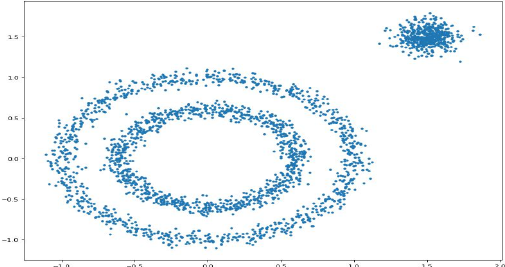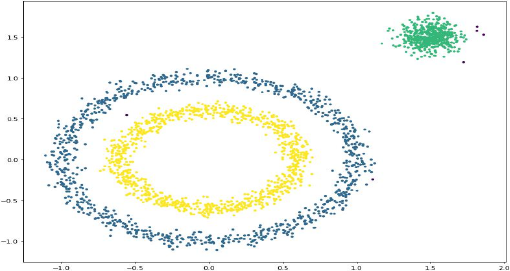3.利用KD树进行优化

KD树（K-Dimensional Tree），是一种分割k维数据空间的数微信_2018年最新新闻网据结构，是二叉搜索树在多维条件下的推广。主要应用于多维空间关键数据的搜索。KD树的介绍见：https://www.jianshu.com/p/ffe52db3e12b，不赘述。

``` 1 import numpy as np
2 import matplotlib.pyplot as plt
3 import math
4 import random
5 from scipy.sp派克_2018年最新新闻网atial import KDTree
6
7 def my-dbscan2(dataSet, eps, minPts):
8     # numpy.ndarray的 shape属性表示矩阵的行数与列数
9     # 行数即表小所有点的个数
10     nPoints = dataSet.shape
11     # (1) 标记所有对象为unvisited
12     # 在这里用一个类vPoints进行实现
13     vPoints = visitlist(count=nPoints)
14     # 初始化簇标记列表C，簇标记为 k
15     k = -1
16     C = [-1 for i in range(nPoints)]
17     # 构建KD-Tree，并生成所有距离<=eps的点集合
18     kd = KDTree(X)
19     while(vPoints.unvisitednum>0):
20         # (3) 随机选择一个unvisited对象p
21         p = random.choice(vPoints.unvisitedlist)
22         # (4) 标t己p为visited
23         vPoints.visit(p)
24         # (5) if p 的\$varepsilon\$-邻域至少有MinPts个对象
25         # N是p的\$varepsilon\$-邻域点列表
26         N = kd.query_ball_point(dataSet[p], eps)
27         if len(N) >= minPts:
28             # (6) 创建个一个新簇C，并把p添加到C
29             # 这里的C是一个标记列表，直接对第p个结点进行赋值
30             k += 1
31             C[p] = k
32             # (7) 令N为p的\$varepsilon\$-邻域中的对象的集合
33             # N是p的\$varepsilon\$-邻域点集合
34             # (8) for N中的每个点p"
35             for p1 in N:
36                 # (9) if p"是unvisited
37                 if p1 in vPoints.unvisitedlist:
38                     # (10) 标记p"为visited
39                     vPoints.visit(p1)
40                     # (11) if p"的\$varepsilon\$-邻域至少有MinPts个点，把这些点添加到N
41                     # 找出p"的\$varepsilon\$-邻域点，并将这些点去重新添加到N
42                     M = kd.query_ball_point(dataSet[p1], eps)
43                     if len(M) >= minPts:
44                         for i in M:
45                             if i not in N:
46                                 N.append(i)
47                     # (12) if p"还不是任何簇的成员，把p"添加到c
48                     # C是标记列表，直接把p"分到对应的簇里即可
49                     if C[p1] == -1
50                         C[p1] = k
51         # (15) else标记p为噪声
52         else:
53             C[p1] = -1
54
55     # (16) until没有标记为unvisited的对象
56     return C```

```1 import time
2 start = time.time()
3 C1 = my_dbscanl(X, 0.1, 10)
4 end = time.time()
5 print "`运行时间`:", end - start
6 plt.scatter(X[:, 0], X[:, 1], c=C1, marker=".")
7 plt.show()
8 >>> `运行时间：`29.1249849796```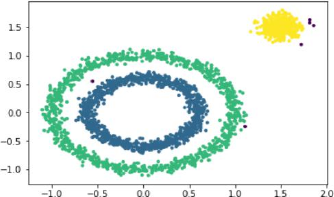```1 import time
2 start = time.time()
3 C2 = my_dbscan2(X, 0.1, 10)
4 end = time.time()
5 print "运行时间:", end - start
6 plt.scatter(X[:, 0], X[:, 1], c=C2, marker=".")
7 plt.show()
8 >>> 运行时间：4.72340583801```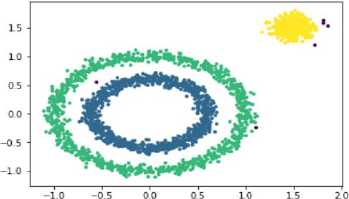4.后记

``` 1 # DBSCAN eps = 0.1, MinPts = 10
2 import time
3 from sklearn.cluster import DBSCAN
4 start = time.time()
5 C = DBSCAN(eps=0.1, min_pts=10).
6 end = time.time()
7 print "运行时间：", end - start
8 plt.scatter(X[:, 0], X[:, 1], c=C, marker=".")
9 plt.show()
10 >>> 运行时间：0.0240921974182```